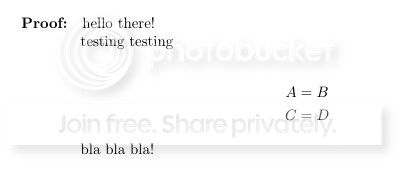# Hanging Indents & troubles with \newenvironment

I'm new to latex, and I've done a lot of searching but can't seem to find a fix for this:

I want a pretty straightforward proof environment, proof followed by a hanging indent.

Like this:

\documentclass{article}
\parindent=0pt
\parskip=\medskipamount
\usepackage{amsthm}
\usepackage{amssymb}
\usepackage{amsmath}

\begin{document}
\paragraph{Proof:}
\hangindent=4em
hello there!\\
testing testing\\
\begin{flalign*}
A &= B \\
C &= D \end{flalign*}
bla bla bla!\\
\end{document}


Which seems to work nicely.But when I try to make a \newenvironment out of it, it doesn't seem to work:

\documentclass{article}
\parindent=0pt
\parskip=\medskipamount
\usepackage{amsthm}
\usepackage{amssymb}
\usepackage{amsmath}

\newenvironment{pf}
{\paragraph{Proof:} \hangindent=4em}{}

\begin{document}
\begin{pf}
hello there!\\
testing testing\\
\begin{flalign*}
A &= B \\
C &= D \end{flalign*}
bla bla bla!\\
\end{pf}
\end{document}


The indent is no longer preserved (the last line is off), and I can't figure out why...

In another more convoluted attempt, I've tried \tabu inside of a \tabu:

\documentclass[12pt,parskip=full]{scrbook}
\usepackage[utf8]{inputenc}
\usepackage{amsthm}
\usepackage{amssymb}
\usepackage{amsmath, tabu}
\usepackage{graphicx}
\usepackage{tabularx}

\newenvironment{pfa}
{
\begin{tabu}{l|p{16cm}}
\textit{Proof:} &
\begin{tabu}{p16cm}
}
{ \qed
\end{tabu}
\end{tabu}
}

\begin{document}
\hrulefill

\begin{tabu}{l|p{16cm}}
Proof: & \begin{tabu}{p{16cm}}
hello there!\\
testing testing\\
{\begin{align}
A &= B \\
C &= D \end{align}}
\qed
\end{tabu}
\end{tabu}

\hrulefill

\begin{pfa}
hello there!\\
{\begin{align}
A &= B \\
C &= D \end{align}}
\end{pfa}

\end{document}


Once more, the manually written version works, but the \newevironment fails and throws errors which I can't seem to fix.

• I think your approach here is somewhat flawed using \hangindent. If you want an entire block of code offset from the margin(s), then you can use adjustwidth from changepage. For more on this, see Indenting a whole paragraph.
– Werner
Aug 24, 2016 at 22:30
• The TeXnical reason why you are experiencing this is a bit difficult to explain to a beginner in 600 characters, but it can be fixed by adding a \par at the end of the environment, like this: \newenvironment*{pf}{\paragraph*{Proof:}\setlength\hangindent{4em}}{\par} (note that I use \paragraph*, not \paragraph). But, as @Werner has already remarked, this is not the right approach. May I also suggest a description environment, customized to your needs with the help of the enumitem package?
– GuM
Aug 24, 2016 at 23:41
• Also, may I very strongly recommend avoiding tabu at all costs? Believe me, you do not want to use this package.
– cfr
Aug 24, 2016 at 23:55

Using a table for the proof is surely wrong, because this will not break across pages. Also \hangindent will not work properly with lists (enumerate or itemize) in the proof.

You can make an easy modification to the proof environment provided by amsthm to use \list instead of \trivlist, thus allowing to set the left margin.

\documentclass{article}
\usepackage{amsmath}
\usepackage{amsthm}

% the following two are just for the example
\usepackage[a4paper,margin=3cm]{geometry}
\usepackage{lipsum}

\makeatletter
\renewenvironment{proof}[\proofname]
{\par
\pushQED{\qed}%
\normalfont
\topsep6\p@\@plus6\p@\relax
\list{}{%
\settowidth\leftmargin{\textbf{#1:}}%
}%
\item[\hskip-\leftmargin\bfseries #1:]\ignorespaces
}
{%
\popQED\endlist\@endpefalse
}
\makeatother

\begin{document}

\lipsum

\begin{proof}
\lipsum*
\begin{align*}
A &= B \\
C &= D
\end{align*}
\lipsum*
\end{proof}

\begin{proof}
\lipsum*
\begin{align*}
A &= B \\
C &= D \qedhere
\end{align*}
\end{proof}

\end{document}• This works nicely! Thank you. I'll take time to look through and try to figure out what each bit means, lots of stuff to learn. Aug 25, 2016 at 20:30

You simply can use the ntheorem package, which defines a \theoremindent length. I patched the nonumberplain theorem style to adapt it to this indention. You can use an optional argument to the environment

Another advantage is that you can have an end-of-proof symbol (that you can choose as you want), which will be automatically placed, even if the proof ends in a displayed equation.

\documentclass{article}
\usepackage[utf8]{inputenc}
\usepackage[T1]{fontenc}
\usepackage{lmodern}
\usepackage{showframe}
\renewcommand\ShowFrameLinethickness{0.2pt}

\parindent=0pt
\parskip=\medskipamount
%\usepackage{amsthm}
\usepackage{amssymb, amsfonts}
\usepackage{amsmath}

\usepackage[thmmarks,  thref, amsmath]{ntheorem}
\makeatletter
\newtheoremstyle{proof}%
\makeatother
\theoremindent=4em
\theoremstyle{proof}
\theorembodyfont{\normalfont}
\theoremseparator{:}
\theoremsymbol{\ensuremath{\square}}
\newtheorem{proof}{\llap{Proof}}

\begin{document}

\begin{proof}
hello there!\\
testing testing
\begin{flalign*}
A &= B \\
C &= D
\end{flalign*}
\end{proof}

\begin{proof}[of some theorem]
hello there!\\
testing testing
\begin{flalign*}
A &= B \\
C &= D
\end{flalign*}
Blah blah blah
\end{proof}

\end{document}• Thanks! At first I would think that \theoremstyle would provide the most flexibility... However, experimenting around with it, I ran into some troubles with existing theorem styles that were dependent on the amsthm package... It seems that all the other theorem types borrow some behaviors of the proof type instead of remaining distinct and separate. That may because I'm not too well versed in theorem styles yet... For example: \theoremstyle{definition} \newtheorem{ex}{definition}{Example}[section] and having that "example" style different then the "proof" style... Aug 25, 2016 at 20:51
• You can't load both amsthm and ntheorem to format your theorem-like structures. However, there is amsthm oprion for ntheorem, which emulates the definitions of amsthm. 2nd point: the syntax for your ex environment would be theoremstyle{plain}\theorembodyfont{\upshape} \newtheorem{ex}{Example}[section]. Each characteristic for a theorem set is valid for all subsequent theorem declarations, until this characteristic is changed. So, for instance ex has to be declared before proof , or you must add \theoremindent=0pt. Aug 25, 2016 at 22:29